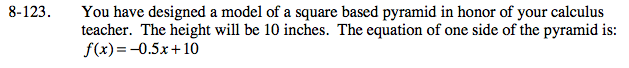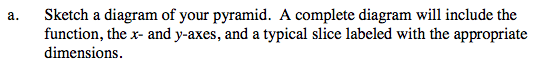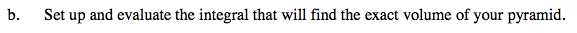### Home > CALC > Chapter 8 > Lesson 8.3.3 > Problem8-123

8-123.
1. You have designed a model of a square based pyramid in honor of your calculus teacher. The height will be 10 inches. The equation of one side of the pyramid is: f(x) = −0.5x + 10 Homework Help ✎

1. Sketch a diagram of your pyramid. A complete diagram will include the function, the x- and y-axes, and a typical slice labeled with the appropriate dimensions.

2. Set up and evaluate the integral that will find the exact volume of your pyramid.Pyramids have pointy tips. If the height is 10 inches, the base would start at y = 0.Notice that the cross-sections are perpendicular to the y-axis, so set up and evaluate an integral in terms of y.

Use the eTool below to help solve the problem.
Click the link at right for the full version of the eTool: Calc 8-123 HW eTool